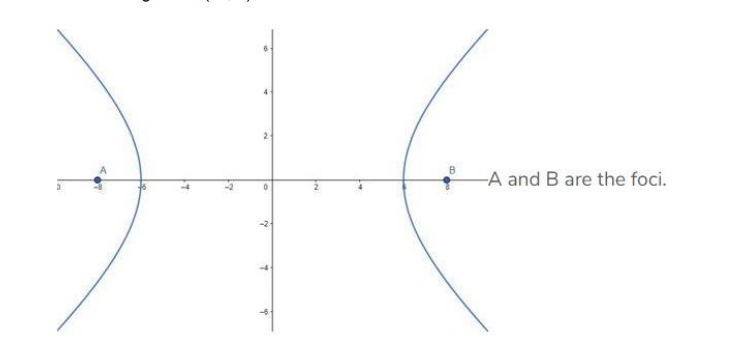# Find the equation of the hyperbola with vertices at (±6, 0) and foci at (±8, 0).`
Question:

Find the equation of the hyperbola with vertices at (±6, 0) and foci at (±8, 0).

Solution:

Given: Vertices at (±6, 0) and foci at (±8, 0)

Need to find: The equation of the hyperbola

Let, the equation of the parabola be:

$\frac{x^{2}}{a^{2}}-\frac{y^{2}}{b^{2}}=1$

Vertices of the parabola is at $(\pm 6,0)$

That means $\mathrm{a}=6$

The foci are given at $(\pm 8,0)$That means, ae = 8, where e is the eccentricity.

$\Rightarrow 6 \mathrm{e}=8[$ As $\mathrm{a}=6]$

$\Rightarrow \mathrm{e}=\frac{8}{6}=\frac{4}{3}$

We know that, $e=\sqrt{1+\frac{b^{2}}{a^{2}}}$

Therefore,

$\Rightarrow \sqrt{1+\frac{b^{2}}{a^{2}}}=\frac{4}{3}$

$\Rightarrow 1+\frac{b^{2}}{a^{2}}=\frac{16}{9}$ [Squaring both sides]

$\Rightarrow \frac{b^{2}}{a^{2}}=\frac{16}{9}-1=\frac{7}{9}$

$\Rightarrow b^{2}=a^{2} \frac{7}{9}$

$\Rightarrow \mathrm{b}^{2}=36 \times \frac{7}{9}=4 \times 7=28[$ As $\mathrm{a}=6]$

So, the equation of the hyperbola is,

$\frac{x^{2}}{a^{2}}-\frac{y^{2}}{b^{2}}=1 \Rightarrow \frac{x^{2}}{36}-\frac{y^{2}}{28}=1$ [Answer]